# Rigid body game physics 2

The following article is heavily based on Hubert Eichner's article on equality constraints. The math for the joint types was taken from Kenny Erleben's PhD thesis.

## Constraint Impulses

A constraint between two objects is specified as a multi-dimensional function C(y(t)). The constrained is fulfilled by attempting to keep each component of the derivative of C at zero:J is the Jacobian matrix and u is a twelve-element vector containing the linear and rotational speed of the two bodies. Here the derivative of the orientation is represented using the rotational speed ω.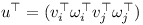The two objects without a connecting joint would together have twelve degrees of freedom. Let's assume that the two objects are connected by a hinge joint. In this case the function C has five dimensions because a hinge only leaves seven degrees of freedom. The Jacobian J has five rows and twelve columns. In practise an additional vector b is used to correct for numerical errors.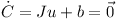The twelve-element constraint impulse P does not do any work and therefore must be orthogonal to u.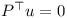The rows of J span the orthogonal space to u. Therefore P can be expressed as a linear combination of the rows of J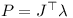where λ is a vector with for example five elements in the case of the hinge joint.

Given an initial estimate for u and the constraint impulse P one can compute the final velocity u.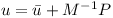M is the twelve by twelve generalised mass matrix which contains the mass and inertia tensors of the two objects.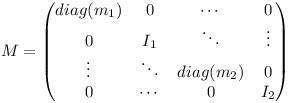Inserting the equation for u into the constraint equation yields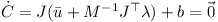This equation can be used to determine λ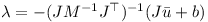λ then can be used to determine the constraint impulse P! When performing the numerical integration, the external forces (multiplied with the timestep) and the constraint impulse are used to determine the change in linear and rotational speed of the two bodies.

## Joints

### Ball-in-Socket Joint

The matrix J for bodies i and j and the velocity vector u can be split up into the linear and angular parts.v is the three-dimensional linear speed and ω is the rotational speed of the individual object. At the anchor point of the joint the speed of the two rigid bodies must be the same:Thus the linear components of J are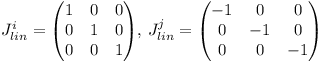and the angular components of J are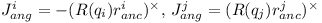where the cross-product matrix of a vector is defined as follows: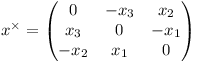The correcting vector b simply corrects for the offset at the anchor point.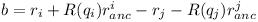### Hinge Joint

A hinge joint has the constraints of the ball-in-socket joint and two additional angular constraints. I.e. there are five constraints altogether.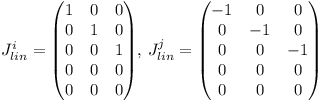The rotation axis in world coordinates can be computed from the average of the two rotated axes which should be coinciding under ideal circumstances.The relative rotation of the two rigid bodies must be parallel to the axis s of the hinge. Given two vectors t1 and t2 orthogonal to the rotation axis of the hinge joint, the two additional constraints are: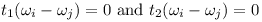Therefore the angular parts of the Jacobian are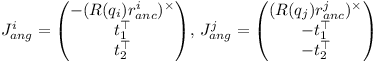The error u of axis misalignment is the cross product of the two rotated axes.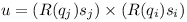The error u is orthogonal to the rotation axis s. It is projected onto the vectors t1 and t2 when computing the correction vector b: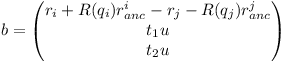See Kenny Erleben's PhD thesis for these and other types of joints (slider joint, hinge-2 joint, universal joint, fixed joint).

## Sequential Impulses

To fulfill multiple constraints, an algorithm called sequential impulses is used. Basically the constraint impulses are updated a few times until the impulses become stable.

• for each iteration
• for each joint
1. compute Jacobian J and correction vector b
2. predict speed u
3. compute λ
4. compute the impulse P
5. add P to accumulated impulses of the two objects
• use impulses and external forces to perform Runge Kutta integration step

The sequential impulse iterations have to be performed four times when using the Runge-Kutta method.

# Rigid body game physics

Inspired by Hubert Eichner's articles on game physics I decided to write about rigid body dynamics as well. My motivation is to create a space flight simulator. Hopefully it will be possible to simulate a space ship with landing gear and docking adapter using multiple rigid bodies.

## The Newton-Euler equation

Each rigid body has a center with the 3D position c. The orientation of the object can be described using a quaternion q which has four components. The speed of the object is a 3D vector v. Finally the 3D vector ω specifies the current rotational speed of the rigid body.

The time derivatives (indicated by a dot on top of the variable name) of the position and the orientation are (Sauer et al.):The multiplication is a quaternion multiplication where the rotational speed ω is converted to a quaternion: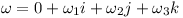In a given time step Δt the position changes as followsThe orientation q changes as shown belowNote that ω again is converted to a quaternion.

Given the mass m and the sum of external forces F the speed changes like thisIn other words this means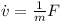Finally given the rotated inertia matrix I(t) and the overall torque τ one can determine the change of rotational speedOr written as a differential equationThese are the Newton-Euler equations. The "x" is the vector cross product. Note that even if the overall torque τ is zero, the rotation vector still can change over time if the inertial matrix I has different eigenvalues.

The rotated inertia matrix is obtained by converting the quaternion q to a rotation matrix R and using it to rotate I₀: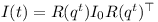The three column vectors of R can be determined by performing quaternion rotations on the unit vectors.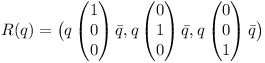Note that the vectors get converted to quaternion and back implicitely.

According to David Hammen, the Newton-Euler equation can be used unmodified in the world inertial frame.

## The Runge-Kutta Method

The different properties of the rigid body can be stacked in a state vector y as follows.The equations above then can be brought into the following form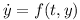Using h=Δt the numerical integration for a time step can be performed using the Runge-Kutta method: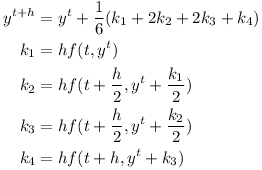The Runge-Kutta algorithm can also be formulated using a function which instead of derivatives returns infitesimal changesThe Runge-Kutta formula then becomesUsing time-stepping with F=0 and τ=0 one can simulate an object tumbling in space.# More minimal speech recognition using Tensorflow

A GRU and a fully connected layer with softmax output can be used to recognise words in an audio stream (sequence to sequence). I recorded a random sequence of 100 utterances of 4 words ("left", "right", "stop", "go") with a sampling rate of 11025Hz. Furthermore 300 seconds of background noise were recorded. All audio data was grouped into chunks of 512 samples. The logarithm of the Fourier spectrum of each chunk was used as input for the GRU. The initial state of the GRU (128 units) was set to zero. The network was trained in a sequence-to-sequence setting to recognise words given a sequence of audio chunks.The example was trained using gradient descent with learning rate alpha = 0.05. The background noise was cycled randomly and words were inserted with random length pause between them. The network was trained to output the word label 10 times after recognising a word. The training took about one hour on a CPU. The resulting example is more minimalistic than the Tensorflow audio recognition example. The algorithm is very similar to an exercise in Andrew Ng's Deep learning specialization.

Update:

# Minimal speech recognition using Tensorflow

An LSTM can be used to recognise words from audio data (sequence to one). I recorded a random sequence of 320 utterances of 5 words ("left", "right", "stop", "go", and "?" for background noise) with a sampling rate of 16kHz. The audio data was split into chunks of 512 samples. The word start and end were identified using a rising and a falling threshold for the root-mean-square value of each chunk. The logarithm of the Fourier spectrum of each chunk was used as input for the LSTM. The initial state and output of the LSTM (16 units each) was set to zero. The final output state of the LSTM was used as input for a fully connected layer with 5 output units followed by a softmax classifier. The network was trained in a sequence-to-one setting to recognise a word given a sequence of audio chunks as shown in the following figure.The example was trained using gradient descent with multiplier alpha = 0.001. The training took seven hours on a CPU. The sequence-to-one setting handles words of different lengths gracefully. The resulting example is more minimalistic than the Tensorflow audio recognition example.

In the following video the speech recognition is used to control a robot.

# A divide-and-conquer implementation of the GJK algorithm

The Gilbert-Johnson-Keerthi (GJK) algorithm is a method for collision detection of convex polyhedra. The method is used in multiple rigid body simulations for computer games.

Given:

• a set of points defining the convex polyhedron A
• a set of points defining the convex polyhedron B

The algorithm returns:

• the distance of the polyhedra
• two closest points
• the two simplices (convex subsets of up to 4 points in 3 dimensions) of A and B containing the two closest points

An n-dimensional simplex is the convex hull of n+1 points as shown in the figure below.The algorithm makes use of the fact, that the distance between two sets A and B is the distance of the Minkowski difference to the origin:whereThe GJK algorithm iteratively updates a simplex until the closest point to the origin is found.

The algorithm iterates using support points. Given a set M and a vector d, the support point is defined as the furthest point of M in direction d: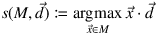The GJK algorithm detects the two closest points of A and B as follows:

1. Choose any point m in M(A,B) in the Minkowski difference of A and B.
2. Set the initial simplex w0 to w0={m}.
3. Let d be the closest point of wk to the origin.
4. If d s(wk, -d)>=d s(M,-d) then return d.
5. Set w'k+1=wk∪{s(M,-d)}
6. Set wk+1 to the smallest simplex in w'k+1 still containing s(M,-d).
7. Continue with step 3.

Note that step 3 requires finding the closest point of the simplex wk to the origin. Most implementations of the GJK algorithm seem to use the following approach:

• Check if the origin is contained in the 3D simplex.
• If not, check whether the origin is near one of the 4 surfaces of the simplex.
• If the origin is not in the Delaunay region of any surface, check whether the origin is near one of the 6 edges.
• If not in a Delaunay region of an edge, find the closest point of the 4 points.

A much more compact implementation can be obtained using a divide-and-conquer approach:

• Let wk={wk0,wk1,...,wkn}
• Solve the least squares equation system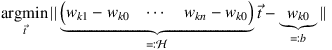• If all ti>=0 and t1+t2+...+tn<=1 (or n=0), then wk0+Ht is the closest point.
• Otherwise take the closest point of all sub-simplices with n-1 dimensions using the approach above (i.e. recursion).

Note that this approach will visit each edge up to two times, and each point up to three times. The performance is not optimal, but it makes for a much more concise implementation.

The least squares solution is: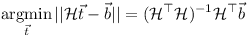Here follows an implementation in Scheme (GNU Guile). By using pairs of points from A and B instead of the Minkowski difference, one can keep track of the information required to determine the pair of closest points of A and B (instead of the closest point of M to the origin).

Here an example of two colliding cuboids simulated using this implementation is shown:

Any feedback, comments, and suggestions are welcome.

Enjoy!

Update:

I noticed that Baraff just uses a separating plane algorithm to achieve the same!